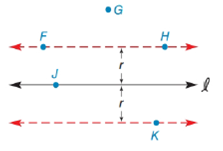Chapter 7.1, Problem 2E### Elementary Geometry for College St...

6th Edition
Daniel C. Alexander + 1 other
ISBN: 9781285195698

#### Solutions

Chapter
Section### Elementary Geometry for College St...

6th Edition
Daniel C. Alexander + 1 other
ISBN: 9781285195698
Textbook Problem
1 views

# Note: Exercises preceded by an asterisk are of a more challenging nature.In the figure, which of the points E ,   F ,   G ,   H , and K belong to “the locus of points in the plane that are at distance r from line l ”?To determine

To find:

The locus points that are in the plane at a distance of r from the line l from the given figure.

Explanation

Definition:

A locus is set of all points and only those points that satisfy a given condition or set of conditions.

In must be noted that, the phrase “all points and only those points” has dual meaning as follows:

1. All points of the locus satisfy the given condition.

2. All points satisfying the given locus conditions are included in the locus.

Calculation:

From the given figure,

We find that dashed line is the locus of points in the plane that are at distance r from line l

### Still sussing out bartleby?

Check out a sample textbook solution.

See a sample solution

#### The Solution to Your Study Problems

Bartleby provides explanations to thousands of textbook problems written by our experts, many with advanced degrees!

Get Started